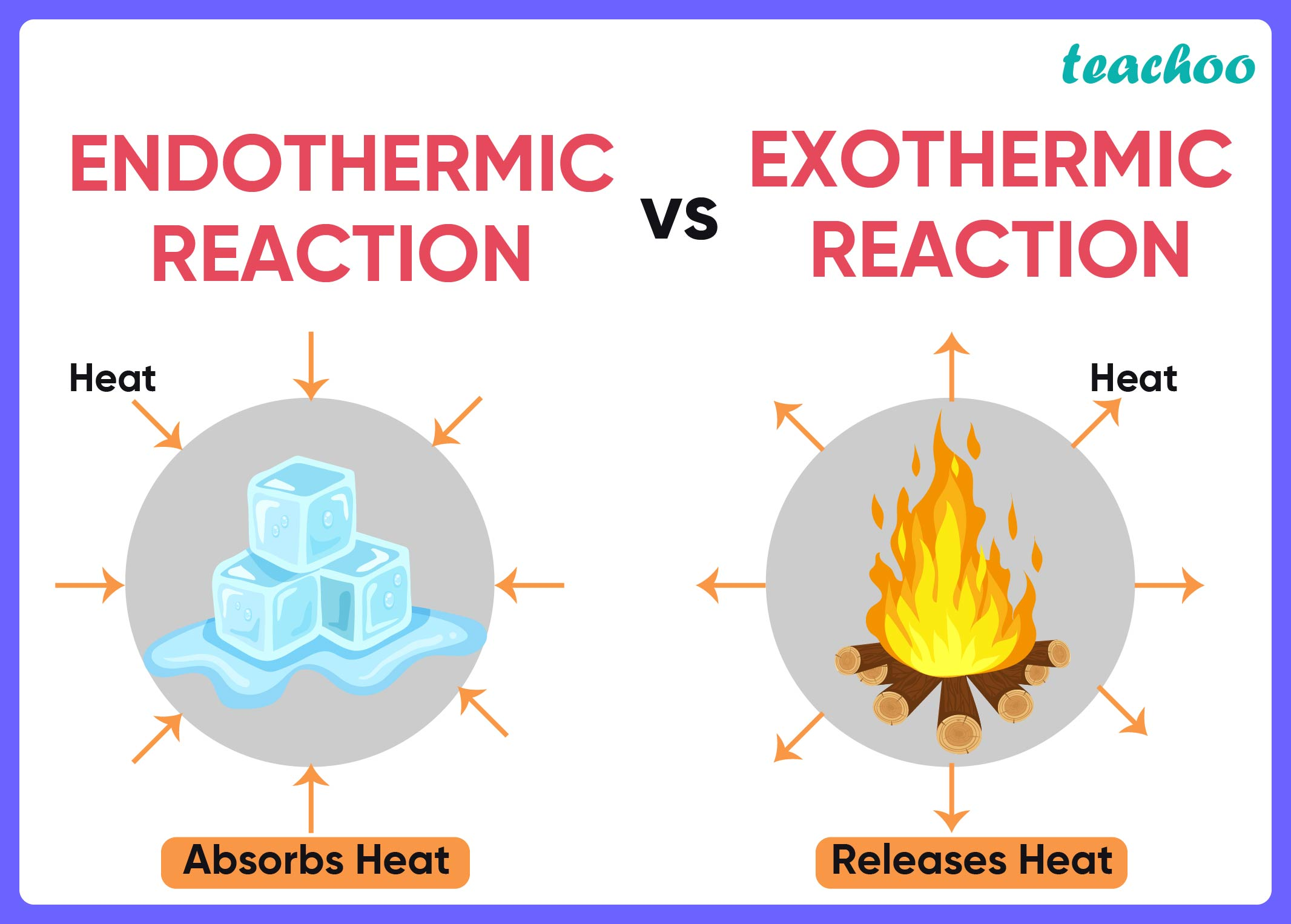MCQs from NCERT Exemplar

Class 10
Chapter 1 Class 10 - Chemical Reactions and Equations

## (D) (ii) and (iv)Here,

• Endo means in and thermic means heat . So, in these reactions heat is absorbed.
• Exo means out and thermic means heat . So, in these reactions, heat is evolved

Here,

• Dilution of sulphuric acid generates heat, so it is an exo thermic process
• Sublimation of dry ice needs heat, so it is an endo thermic process
• Condensation of water vapours generates heat, so it is an exo thermic process
• Evaporation of water needs heat, so it is an endo thermic process

So, the correct answer is (d) -  (ii) and (iv)

Learn in your speed, with individual attention - Teachoo Maths 1-on-1 Class